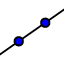# Exploring Reflections 2

## Question 2

Find the equation for the line of reflection that places point C' at (0,1), B' at (-1,0), and A' at (-3,3).

## Question next

After you are sure you have Question 2 correct, draw a lineconnecting each of the points on the preimage to their corresponding points on the image (ie, connect A to A', B to B', and C to C'). What is the slope of the lines you have drawn? What is the relationship of these lines to the line of reflection? Hint, look at the angle between the line of reflection and any line that you drew. You can do this by selecting the angletool and then clicking the two lines you'd like the angle between.

Move on the Exploring Reflections 3.